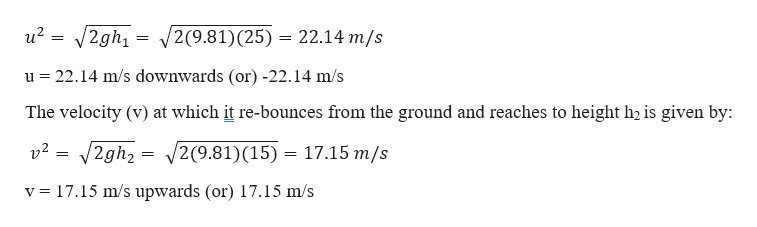# After falling from rest from a height of 25 m, a 0.30-kg ball rebounds upward, reaching a height of 15 m. If the contact between ball and ground lasted 2.1 ms, what average force was exerted on the ball? (Enter the magnitude.)

Question

After falling from rest from a height of 25 m, a 0.30-kg ball rebounds upward, reaching a height of 15 m. If the contact between ball and ground lasted 2.1 ms, what average force was exerted on the ball? (Enter the magnitude.)

check_circleExpert Solution
Step 1

Given information:

The mass of the ball (m) = 0.3 kg

Height from which it falls (h1) = 25 m

Height to which it rebounds (h2) = 15 m

The time for which the force acts (Δt) = 2.1 ms

Consider the down ward direction as “-ve” and upward direction as “+ve”

Step 2

The velocity (u) at which it reaches the ground wh...help_outlineImage Transcriptioncloseu2 = 2gh1 2(9.81) (25) = 22.14 m/s = u 22.14 m/s downwards (or) -22.14 m/s The velocity (v) at which it re-bounces from the ground and reaches to height h2 is given by: /2gh2 = 2(9.81) (15) 17.15 m/s v2 17.15 m/s upwards (or) 17.15 m/s v fullscreen

### Want to see the full answer?

See Solution

#### Want to see this answer and more?

Solutions are written by subject experts who are available 24/7. Questions are typically answered within 1 hour*

See Solution
*Response times may vary by subject and question
Tagged in

### Physics